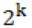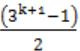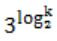## GATE 2002

 Question 1

The rank of the matrixis

 A 4 B 2 C 1 D 0
Question 1 Explanation:
Number of non-zero rows is the rank of the matrix.
 Question 2

The trapezoidal rule for integration give exact result when the integrand is a polynomial of degree:

 A 0 but not 1 B 1 but not 0 C 0 or 1 D 2
Question 2 Explanation:
If curve is of function of degree 1, then curve will be a straight line, and in that case also, all trapezoids will fit completely. So there is no error in degree 1.
 Question 3

The solution to the recurrence equation T(2k) = 3 T(2k-1) + 1, T(1)=1, is:

 ABCDQuestion 3 Explanation:
T(2k) = 3T(2k-1) + 1
T(1)=1
k=0; T(1) = 3T(2-1)+1
k=1; T(2) = 3T(20)+1 = 3(1)+1 = 4
k=2; T(4) = 3T(21)+1 = 3(4)+1 = 13
k=3; T(8) = 3T(22)+1 = 3(13)+1 = 40
k=4; T(16) = 3T(23)+1 = 3(40)+1 = 121
Simply go through the options.
Option B:
k=4 ⇒ (34+1-1)/2
⇒ (243-1)/2
⇒ 121
 Question 4

The minimum number of colours required to colour the vertices of a cycle with η nodes in such a way that no two adjacent nodes have the same colour is

 A 2 B 3 C 4 D n - 2[n/2] + 2
Question 4 Explanation:
We need 2 colours to colour even cycle and 3 colours to colour odd cycle.
 Question 5
In the worst case, the number of comparisons needed to search a singly linked list of length n for a given element is
 A log n B n/2 C (log2)n - 1 D n
Question 5 Explanation:
Worst case time complexity of singly linked list is O(n). So we need n number of comparisons needed to search a singly linked list of length n.
 Question 6

Which of the following is true?

 A The set of all rational negative numbers forms a group under multiplication. B The set of all non-singular matrices forms a group under multiplication. C The set of all matrices forms a group under multiplication. D Both B and C are true.
Question 6 Explanation:
A group G should follow 4 properties:
a. Closure: result of a * b must be in group G.
b. There must be an identity element i.e. e * a = a * e = a
c. There must be an inverse element b for every element a such that a * b = b * a = e
d. Associativity i.e. (a * b) * c = a * (b * c)
Rational negative numbers don't form a group under multiplication, as multiplying two negative numbers results into a positive number, so closure property is not satisfied.
Set of non-singular matrices forms a group under multiplication.
The set of all matrices doesn't form a group under multiplication, since there may not be an inverse for a matrix (in particular, for singular matrices).
 Question 7

The language accepted by a Pushdown Automaton in which the stack is limited to 10 items is best described as

 A Context free B Regular C Deterministic Context free D Recursive
Question 7 Explanation:
Push down automata accept context free grammars but here the value of stack is limited 10 then it accepts regular languages.
 Question 8

“If X then Y unless Z” is represented by which of the following formulas in prepositional logic? (“¬“, is negation, “∧” is conjunction, and “→” is implication)

 A (X∧¬Z)→Y B (X∧Y)→¬Z C X→(Y∧¬Z) D (X→Y)∧¬Z
Question 8 Explanation:
"If X then Y unless Z" ⇒ ¬Z → (X→Y)
⇒ Z ∨ ¬X ∨ Y
⇒ ¬X ∨ Z ∨ Y
Option A:
(X ∧ ¬Z) → Y = ¬(X ∧ ¬Z ) ∨ Y = ¬X ∨ Z ∨ Y Hence, option (A) is correct.
 Question 9

A device employing INTR line for device interrupt puts the CALL instruction on the data bus while

 AB HOLD is active C READY is active D None of the above
Question 9 Explanation:
INTR is a signal which if enabled then microprocessor has interrupt enabled it receives high INR signal & activates INTA signal, so another request can’t be accepted till CPU is busy in servicing interrupt.
 Question 10

In 8085 which of the following modifies the program counter?

 A Only PCHL instruction B Only ADD instructions C Only JMP and CALL instructions D All instructions
Question 10 Explanation:
PCHL Instruction: Which can copy the content from H& L to PC.
ADD Instruction: increments the program counter.
JMP & CALL: Change the values of PC.
 Question 11

In serial data transmission, every byte of data is padded with a ‘0’ in the beginning and one or two ‘1’s at the end of byte because

 A Receiver is to be synchronized for byte reception B Receiver recovers lost ‘0’s and ‘1’s from these padded bits C Padded bits are useful in parity computation D None of the above
Question 11 Explanation:
‘0’ is added at the beginning which can represent receiver about arrival of data and ‘1’ is added at the end which represent receiver state of new arrival of data.
 Question 12

Minimum sum of product expression for f(w,x,y,z) shown in Karnaugh-map below isA xz+y'z B xz'+zx' C x'y+zx' D None of the above
Question 12 Explanation:⇒ xz' + zx'
 Question 13

Which of the following is not a form of memory?

 A instruction cache B instruction register C instruction opcode D translation look-a-side buffer
Question 13 Explanation:
Opcode is the portion of a machine language instruction that specifies the operation to be performed.
 Question 14

The decimal value 0.25

 A is equivalent to the binary value 0.1 B is equivalent to the binary value 0.01 C is equivalent to the binary value 0.00111… D cannot be represented precisely in binary
Question 14 Explanation:
1st Multiplication iteration:
Multiply 0.25 by 2.
0.25×2 = 0.50 (product)
Fractional part = 0.50
Carry = 0
2nd Multiplication iteration:
Multiply 0.50 by 2.
0.50×2 = 1.00 (product)
Fractional part = 0.00
Carry = 1
The fractional part in the 2nd iteration becomes zero and so we stop the multiplication iteration.
Carry from 1st multiplication iteration becomes MSB and carry from 2nd iteration becomes LSB. So the result is 0.01.
 Question 15

The 2’s complement representation of the decimal value -15 is

 A 1111 B 11111 C 111111 D 10001
Question 15 Explanation:
15 = 1111
-15 = 11111
1's complement = 10000
2's complement = 10001
 Question 16

Sign extension is a step in

 A floating point multiplication B signed 16 bit integer addition C arithmetic left shift D converting a signed integer from one size to another
Question 16 Explanation:
Sign extension is a step in converting a signed integer from on size to another.
 Question 17

In the C language

 A At most one activation record exists between the current activation record and the activation record for the main B The number of activation records between the current activation record and the activation record for the main depends on the actual function calling sequence. C The visibility of global variables depends on the actual function calling sequence. D Recursion requires the activation record for the recursive function to be saved on a different stack before the recursive fraction can be called.
Question 17 Explanation:
In C Language a function can create activation record from the created function stack.
There are 17 questions to complete.

Register Now